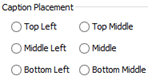# Control Shape Caption Placement using Open APIWhen creating diagrams sometimes you might want to have the caption not showing in the default location, i.e. to have better layout for the containing elements while not having the name being covered. In this case you may adjust the caption position to reserve space for the child elements. To do this you can specify the caption placement of the individual element via the user interface. But instead of manually specify the caption placement shape by shape, you can also do this via the Open API. In this article we will use Component as sample to demonstrate how to control its caption position with Visual Paradigm’s Open API.

## Look up the Component Diagram or create one

We first have to lookup the component diagram in our project. In case the diagram we looking for does not exist we then create one. We create a method to lookup the diagram in our project:

```private IComponentUIModel getComponentFromDiagram() {
// obtain the project and loop through the diagrams
IProject project = ApplicationManager.instance().getProjectManager().getProject();// loop through the diagrams to locate
// the one call "Caption Placement Test"
IDiagramUIModel[] diagrams = project.toDiagramArray();
if (diagrams != null) {
for (IDiagramUIModel diagram : diagrams) {
if (diagram instanceof IComponentDiagramUIModel && "Caption Placement Test".equals(diagram.getName())) {
// once the diagram was found, then see does
// it contain a component call "MyComponent"
IDiagramElement[] elements = diagram.toDiagramElementArray();
if (elements != null) {
for (IDiagramElement element : elements) {
// return the diagram if "MyComponent" was found
if (element instanceof IComponentUIModel && "MyComponent".equals(element.getModelElement().getName())) {
return (IComponentUIModel) element;
}
}
}
}
}
}
return createComponent();
}```

To create the component diagram with the component we will work on:

```public IComponentUIModel createComponent() {
DiagramManager diagramManager = ApplicationManager.instance().getDiagramManager();
// create component diagram
IComponentDiagramUIModel diagram = (IComponentDiagramUIModel) diagramManager.createDiagram(IDiagramTypeConstants.DIAGRAM_TYPE_COMPONENT_DIAGRAM);
diagram.setName("Caption Placement Test");
// create component shape
IComponent componentModel = IModelElementFactory.instance().createComponent();
componentModel.setName("MyComponent");
IComponentUIModel componentShape = (IComponentUIModel) diagramManager.createDiagramElement(diagram, componentModel);
componentShape.setBounds(100, 100, 500, 400);
componentShape.setRequestResetCaption(true);  // show up the diagram
diagramManager.openDiagram(diagram);

// return the component shape
return componentShape;
}```

## Change caption location

If the diagram and the target component was found, then pressing the plugin button will change its caption position. To do this we first obtain all the alignment settings:

```// array of possible caption alignment position
private int[] _alignments = {IShapeUIModel.MODEL_ELEMENT_NAME_ALIGNMENT_ALIGN_TOP_LEFT,
IShapeUIModel.MODEL_ELEMENT_NAME_ALIGNMENT_ALIGN_TOP_MIDDLE,
IShapeUIModel.MODEL_ELEMENT_NAME_ALIGNMENT_ALIGN_TOP_RIGHT,
IShapeUIModel.MODEL_ELEMENT_NAME_ALIGNMENT_ALIGN_MIDDLE_LEFT,
IShapeUIModel.MODEL_ELEMENT_NAME_ALIGNMENT_ALIGN_MIDDLE,
IShapeUIModel.MODEL_ELEMENT_NAME_ALIGNMENT_ALIGN_MIDDLE_RIGHT,
IShapeUIModel.MODEL_ELEMENT_NAME_ALIGNMENT_ALIGN_BOTTOM_LEFT,
IShapeUIModel.MODEL_ELEMENT_NAME_ALIGNMENT_ALIGN_BOTTOM_MIDDLE,
IShapeUIModel.MODEL_ELEMENT_NAME_ALIGNMENT_ALIGN_BOTTOM_RIGHT};```

After that we obtain the component and retrieve its current alignment setting and match it with our array. After that we advance the setting by one and set it back to the component.

```// try to obtain the component diagram from project, or create one if not found
IComponentUIModel componentShape = getComponentFromDiagram();
if (componentShape != null) {
// obtain the original name alignment value
int alignment = componentShape.getModelElementNameAlignment();
// if alignment is set to follow diagram, then change it as top left
if (alignment == IShapeUIModel.MODEL_ELEMENT_NAME_ALIGNMENT_FOLLOW_DIAGRAM) {
alignment = IShapeUIModel.MODEL_ELEMENT_NAME_ALIGNMENT_ALIGN_BOTTOM_RIGHT;
}
// lookup the index of current alignment value
// in _alignments array, and increase index by 1
for (int i = 0; i < _alignments.length; i++) {
if (alignment == _alignments[i]) {
alignment = i+1;
break;
}
}
// if alignment value is bigger then the length of
// _alignments array, then use mod to calculate the
// reminder value as the new index
if (alignment >= _alignments.length) {
alignment = _alignments.length % alignment;
}

// set the new name alignment
componentShape.setModelElementNameAlignment(_alignments[alignment]);```

## Sample Plugin

The sample plugin attached in this article demonstrate how to change the caption placement using Open API. After you deploy the plugin into Visual Paradigm, you can click the plugin button in application toolbar to create the Component Diagram.

If the Component Diagram already created then press the button again will change the caption placement of the component inside the diagram.